# Game Theory With Apache Spark, Part 4

### We wrap up this interesting look into game theory and Apache Spark by finalizing the algorithms and Java code we started last time.

· Big Data Zone · Tutorial
Save
5.22K Views

## Series So Far

### Bringing Pieces Together: Example 1

Now let us revisit Example 1 from the previous article and employ Part 1 and 2 algorithms to obtain the results in Table 3. We start with constructing variables `Ul` and `consumptionSets` that correspond to the rows U and L in Table 2 for each agents 1 - 4.

``````private static void Example1(){
double cons_set1_vals[] = {0,8,4,2,8,4,2,8,4};
DenseVector consSet1 = new DenseVector(cons_set1_vals);

double u1_vals[] = {0,2,3,5,2,3,6,3,6};
DenseVector u1 = new DenseVector(u1_vals);

double cons_set2_vals[] = {4,4,0,0,8,6,0,4,2};
DenseVector consSet2 = new DenseVector(cons_set2_vals);

double u2_vals[] = {2,3,0,0,2,4,0,3,5};
DenseVector u2 = new DenseVector(u2_vals);

double cons_set3_vals[] = {1,2,4,2,4,2,1,8,4};
DenseVector consSet3 = new DenseVector(cons_set3_vals);

double u3_vals[] = {1,2,3,3,1,2,3,1,1};
DenseVector u3 = new DenseVector(u3_vals);

double cons_set4_vals[] = {2,1,0,0,2,1,0,1,0};
DenseVector consSet4 = new DenseVector(cons_set4_vals);

double u4_vals[] = {5,2,0,0,3,2,0,4,0};
DenseVector u4 = new DenseVector(u4_vals);

ArrayList<Vector> Ul = new ArrayList<Vector>();

ArrayList<Vector> consumptionSets = new ArrayList<Vector>();
...``````

Then, we define S, the available supply units from Table 1.

``````    double S_vals[] = {4,2,1,1,4,4,1,4,2};
DenseVector S = new DenseVector(S_vals);
...``````

Finally, we call `getOptimalAssignmentsAndPrice()` to obtain the optimal price and corresponding assignments. We then pass those values to  `getAllocations()` to obtain the allocations corresponding to the optimal price.

``````AssignmentsAndPrice Optimal = getOptimalAssignmentsAndPrice(Ul, S,
consumptionSets);
getAllocations(Optimal.price, Ul, S, consumptionSets, Optimal.assignmentsL);
}``````

We placed a breakpoint in the last statement in  `getAllocations()`, i.e. next to return allocations, to view the variables via the debugger. That gives us the results in Table 3.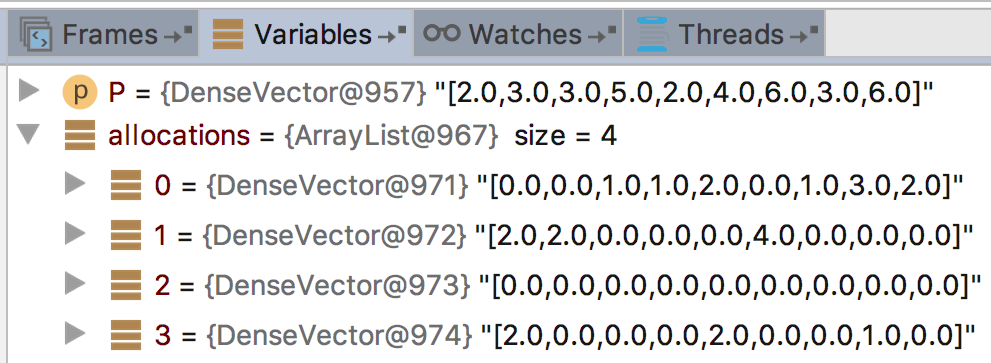Figure 4. Variables in the debugger that show optimal price and allocations for Example 1.

### Bringing Pieces Together: Example 2

Let us revisit Example 2 from the previous article and employ Part 1 and 2 algorithms to obtain the results in Table 5. We start with constructing variables  `Ul` and  `consumptionSets` that correspond to the rows U and L in Table 4 for each agents 1 - 4.

``````private static void Example2_Step1(){

double cons_set1_vals[] = {50,20,30,100,0,0,0,0,0,0,0};
DenseVector consSet1 = new DenseVector(cons_set1_vals);

double u1_vals[] = {3,3,0,6,0,0,0,0,0,0,0};
DenseVector u1 = new DenseVector(u1_vals);

double cons_set2_vals[] = {40,40,20,50,0,0,0,0,0,0,0};
DenseVector consSet2 = new DenseVector(cons_set2_vals);

double u2_vals[] = {5,5,0,8,0,0,0,0,0,0,0};
DenseVector u2 = new DenseVector(u2_vals);

double cons_set3_vals[] = {0,0,0,0,20,20,10,50,0,0,0};
DenseVector consSet3 = new DenseVector(cons_set3_vals);

double u3_vals[] = {0,0,0,0,5,5,5,5,0,0,0};
DenseVector u3 = new DenseVector(u3_vals);

double cons_set4_vals[] = {0,0,50,0,80,30,40,50,100,100,50};
DenseVector consSet4 = new DenseVector(cons_set4_vals);

DenseVector u4 = (Vectors.zeros(11)).toDense();

ArrayList<Vector> Ul = new ArrayList<Vector>();

ArrayList<Vector> consumptionSets = new ArrayList<Vector>();
...``````

Then, we define S, the available supply units from Table 4.

``````    double S_vals[] = {50,50,100, 50, 100, 50, 50, 100, 100, 100, 50};
DenseVector S = new DenseVector(S_vals);
...``````

Finally, we call  `getOptimalAssignmentsAndPrice()` to obtain the optimal price and corresponding assignments. We then pass those values to `getAllocations()` to obtain the allocations corresponding to the optimal price.

``````    AssignmentsAndPrice Optimal = getOptimalAssignmentsAndPrice(Ul, S,
consumptionSets);
getAllocations(Optimal.price, Ul, S, consumptionSets, Optimal.assignmentsL);
}``````

We placed a breakpoint in the last statement in `getAllocations()`, i.e. next to return allocations, to view the variables via debugger. That shows us below giving the results in Table 5.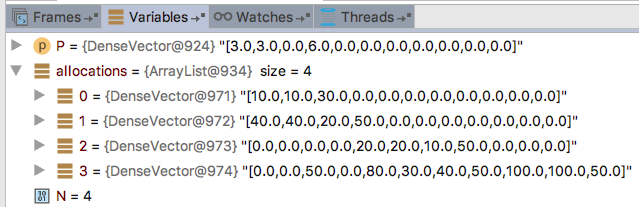Figure 5. Variables in the debugger that show the optimal price and allocations for Example 2, step 1.

Goods are transported based on the above allocation to achieve the state summarized in the below figure, where the number of goods transported over each link is highlighted in red.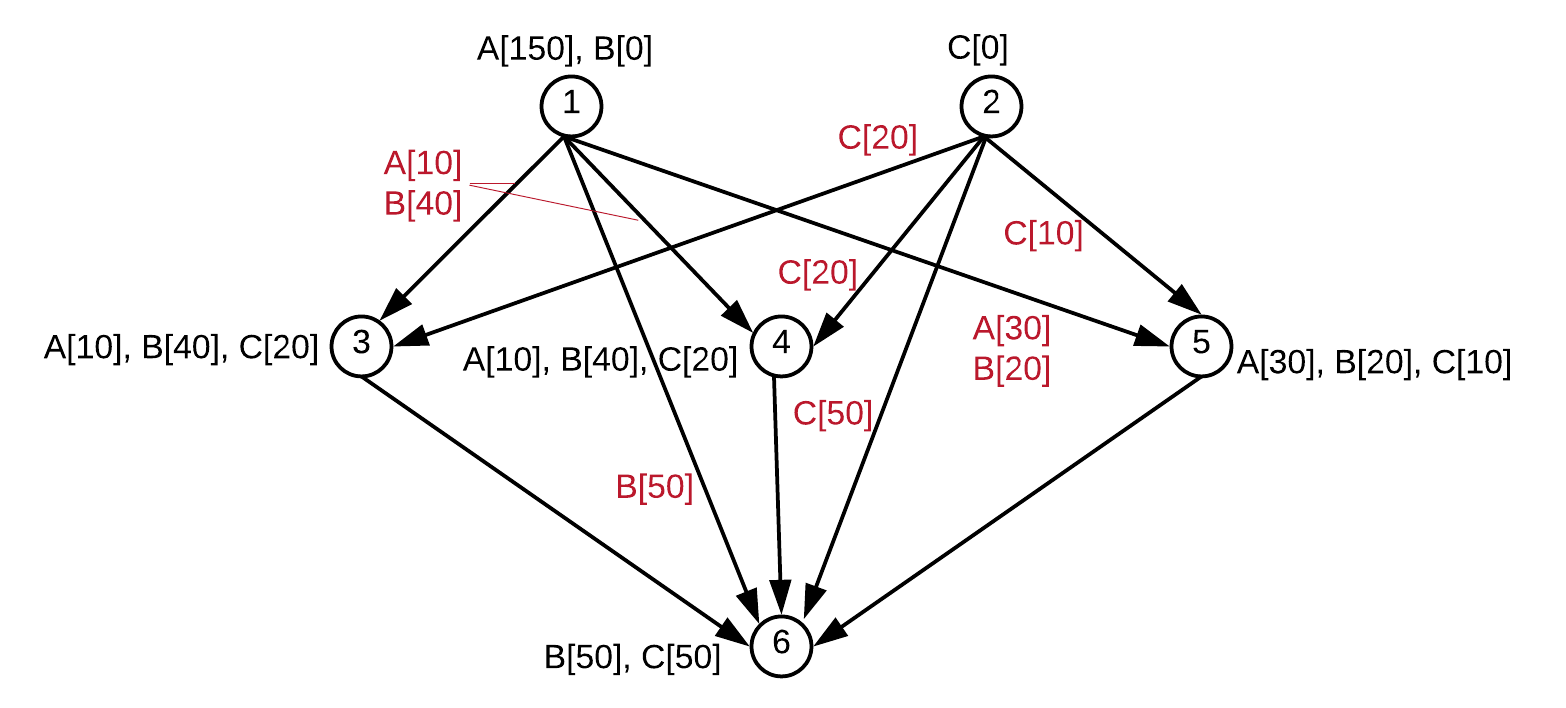Figure 6. Transportation of goods after step 1 is completed. (Copy from Figure 2.)

For step 2, a new formulation is created as shown below. For agent 2, the values for each transportation link is greater than those for agents 1, 3, because the shipping of B type goods has higher priority (5 vs 4).

Table 7

In terms of our code, information in Table 7 is utilized similarly to the previous step.

``````private static void Example2_Step2(){

double cons_set1_vals[] = {0,0,100, 50,0,0,0,0,10,10,30};
DenseVector consSet1 = new DenseVector(cons_set1_vals);

double u1_vals[] = {0,0,4,4,0,0,0,0,4,4,4};
DenseVector u1 = new DenseVector(u1_vals);

double cons_set2_vals[] = {0,0,0,0,0,0,0,0,40,40,20};
DenseVector consSet2 = new DenseVector(cons_set2_vals);

double u2_vals[] = {0,0,0,0,0,0,0,0,5,5,5};
DenseVector u2 = new DenseVector(u2_vals);

double cons_set3_vals[] = {0,0,0,0,0,0,0,0,20,20,10};
DenseVector consSet3 = new DenseVector(cons_set3_vals);

double u3_vals[] = {0,0,0,0,0,0,0,0,4,4,4};
DenseVector u3 = new DenseVector(u3_vals);

double cons_set4_vals[] = {50,50,0,0,100,50,50,100,30,30,0};
DenseVector consSet4 = new DenseVector(cons_set4_vals);

DenseVector u4 = (Vectors.zeros(11)).toDense();

ArrayList<Vector> Ul = new ArrayList<Vector>();

ArrayList<Vector> consumptionSets = new ArrayList<Vector>();

double S_vals[] = {50,50,100,50,100,50,50,100,100,100,50};
DenseVector S = new DenseVector(S_vals);

AssignmentsAndPrice Optimal = getOptimalAssignmentsAndPrice(Ul, S,
consumptionSets);
getAllocations(Optimal.price, Ul, S, consumptionSets, Optimal.assignmentsL);

}``````

The debug point shows us the final results as follows.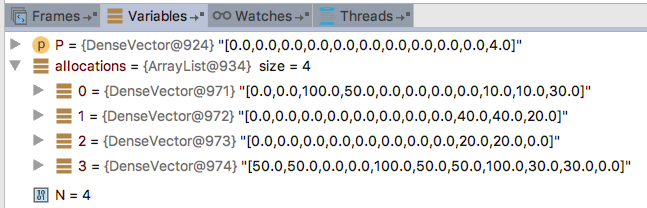Figure 7. Variables in the debugger that show the optimal price and allocations for Example 2, step 2.

Hence, the algorithm yields the following optimal price and allocations.

Table 8

Goods are transported based on the above allocation to achieve the state summarized in the below figure, where the number of goods transported over each link is highlighted in red.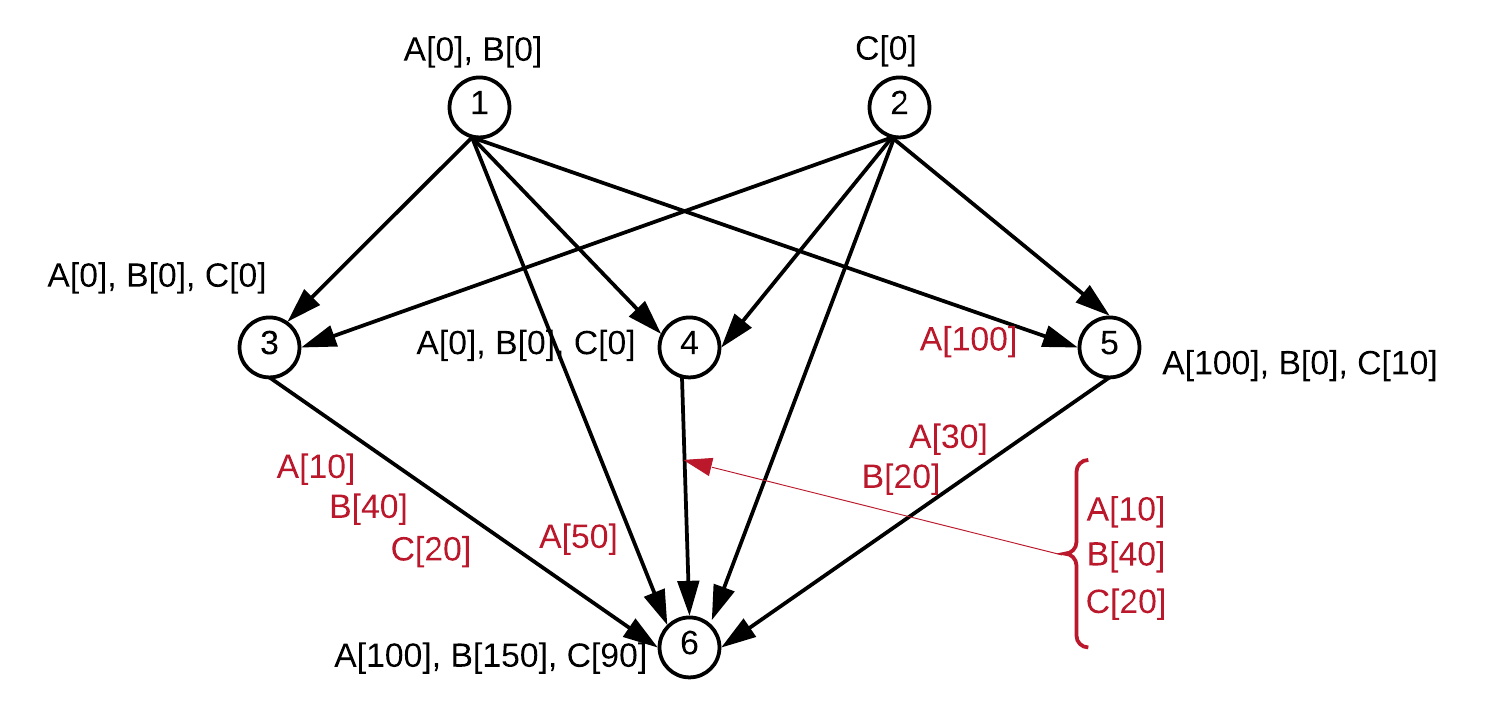Figure 8. Transportation of goods after step 2 is completed.

At this point, all type B goods have been transported. The process is repeated three more times to complete transportation of type A and C goods. Those steps (3 - 5) are shown below (for simplicity we omitted the coding details as those are similar to the code above).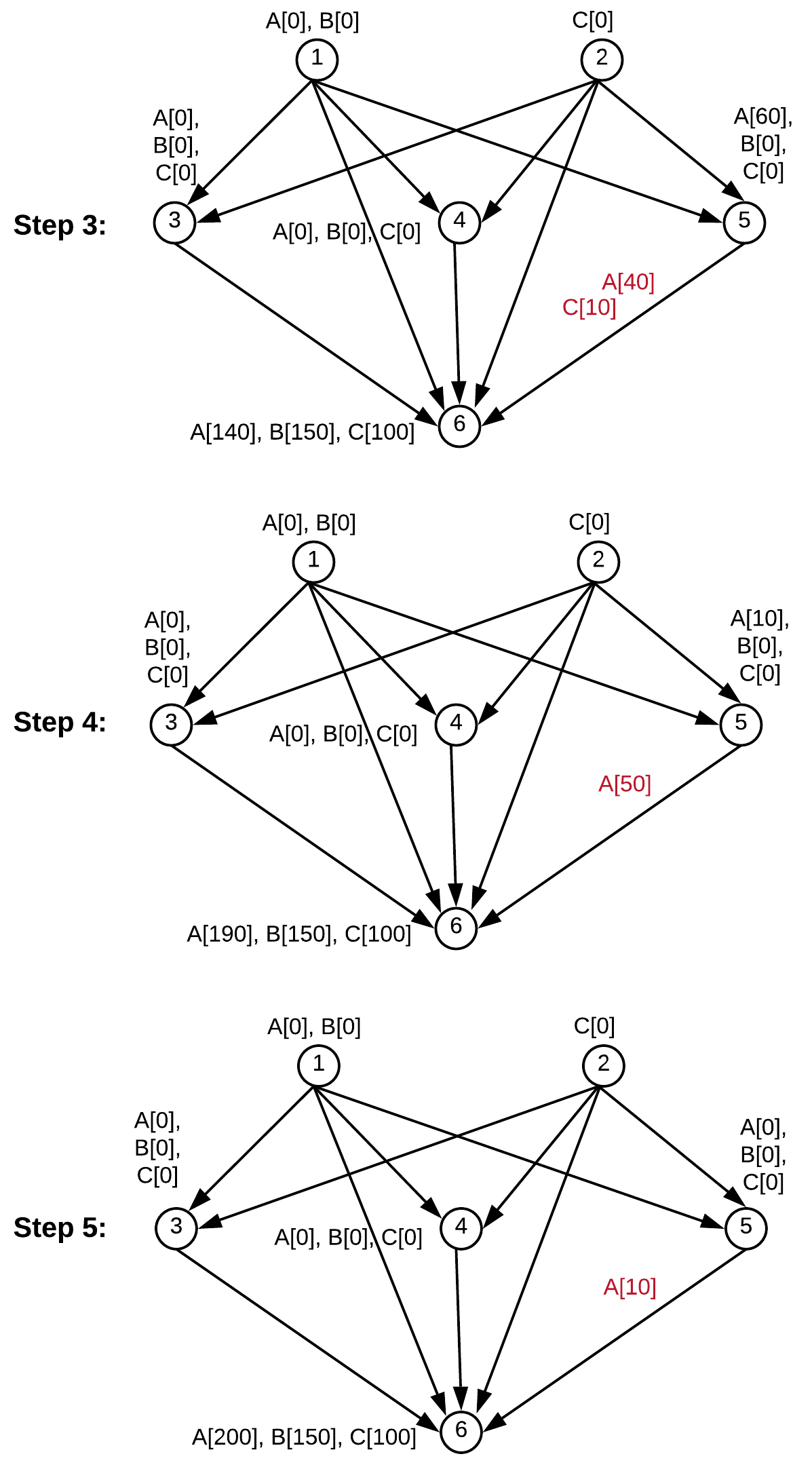Figure 9. Transportation of goods after steps 3 - 5 are completed.

## Conclusion

In this article, we discussed an algorithm based on game theory for optimal resource allocation. The algorithm provides a fairness-based equilibrium where every agent (bidder) maximizes its utility and the resource manager (auctioneer) maximizes the price of the resources it is allocating. In addition, all the available units are allocated across all resource types and no agent is forced to take more than it is willing to. The algorithm is based on economist Ausubel's Efficient Dynamic Auction Method.

We showed via two examples that the algorithm can be applied to different types of resource allocation problems. In one example, we applied the algorithm to allocate cloud computing resources, e.g. CPU, memory, bandwidth, to computing clients. Secondly, we applied the algorithm to a logistics example where various types of goods are transported over shared transportation resources.

We provided an implementation of the algorithm using Apache Spark Machine Learning Library and reviewed the corresponding Java code. Apache Spark Machine Learning Library is particularly useful to implement the algorithm because the corresponding Java API has built-in vector operations, which are essential to execute the algorithm.

In various respects, the dynamic auction method in Ausubel's original paper is more extensive than the version we considered in this article. Firstly, in this article we restricted the value function Ui(x) to be a linear function over x. However, the original algorithm does not have that restriction. Instead, a broader class of concave functions are allowed. Our restriction is for simplicity but also we believe that a linear value function is still very potent in many practical applications (interested users can refer to this article for a review of various concave functions that can be used as a value function in the original paper). Secondly, the original algorithm to find the optimal price does not necessarily start from an initial price vector at 0. However, to deal with an initial price vector different from 0, the algorithm has a descending section as well as an ascending one and hence it is more complex. By restricting the initial price vector to be 0 our implementation became simpler. Thirdly, for the purposes of coherence, we divided the algorithm into parts 1 and 2. Although those concepts are inherent the original algorithm does not have an explicit breakup in that manner.

Topics:
big data, apache spark, game theory, tutorial, data science

Opinions expressed by DZone contributors are their own.